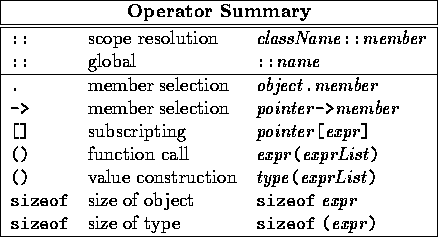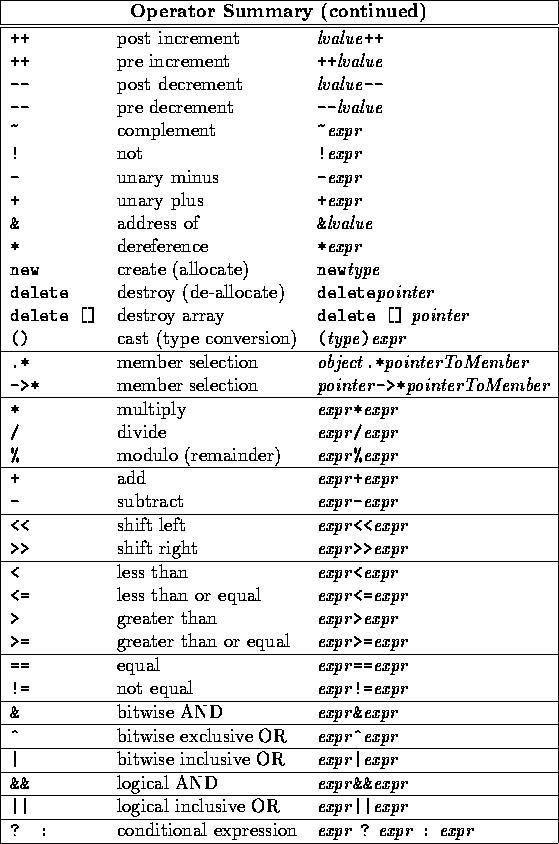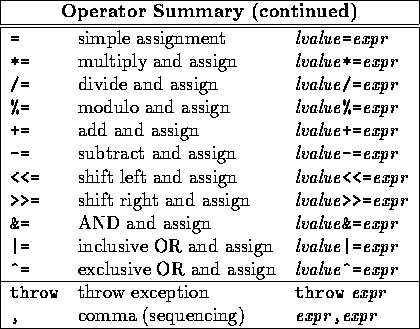# Review and Reinforcement: Operators

Tom Kelliher, CS18

February 7, 1996

# Nested Loops

(Finishing from last time)

Arbitrary nesting possible

Example:

```i = j = 0;
for (i = 0; i < 10; i++) {
cout << setw(10) << i << j << endl;   // first 12 values printed?

for (j = 9; j >= 0; j--)
cout << setw(10) << i << j << endl;
}
```

How could we break out of both loops in last example?

# Operators

Includes:

• Arithmetic
• Relational
• Equality, inequality
• Assignment
• Logical

## Two Somewhat Obscure Operators

Comma ( ,) binary operator:

```for (sum = 0, i = 0; i < MAX; i++)
sum += data[i];

cout << sum << endl;
```

Not the comma separating items in a list (arguments, identifiers in a declaration)

Conditional ( ? :) trinary operator:

```max = (i > j) ? i : j;   // condition parenthesized by convention
```
Equivalent to:
```if (i > j)
max = i;
else
max = j;
```

Another example:

```cout << i << "item" << ((i > 0) ? "s" : "") << endl;
```

Why the parentheses around the entire conditional operator?

## There is No Assignment Statement

Assignment, being an operator, yields a value

All sequential statements (excepting void functions) have/return a value, usually discarded

Consider:

```i = j = 0;

i = (j = 5) + 6;

if ((objectPointer = (object*) malloc(sizeof(object)) == NULL)
fatal("Memory allocation failure");
```

## Order of Operator Evaluation

Affected by:

1. Operator precedence
2. Operator associativity

Assignment, unary operators right associate; all others left associate

Parentheses change the order of evaluation## Expressions Types and Conversions

How can we fix it?

What is the type of 2.5 + 3?

How could we fix this:

```int i = 2;
int j = 5;
float x;

x = 2 / 5;   // x == 0.0
```

In a mixed-type expression, how should we convert types?

Thomas P. Kelliher
Tue Feb 6 10:21:26 EST 1996
Tom Kelliher